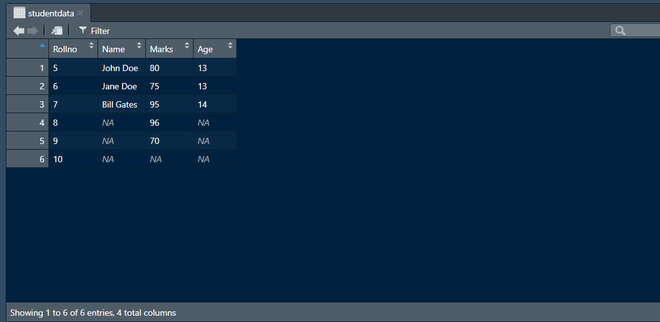# Combine vectors or DataFrames of unequal length in R

• Last Updated : 07 Apr, 2021

In this article, we are going to see how to combine vectors or DataFrames of unequal length into one DataFrame in R Programming Language.

Functions Used:

1. c(“Value1”, “Value2”, “Value3”) : This is a generic function which combines its arguments. The default method combines its arguments(Values) to form a vector.
2. length(vector): Get or set the length of the Vectors, Factors, or any other R Objects.
3. max(…): Returns the maximum of all the values passing inside it’s an argument.
4. rep(x, …): Replicates the values in x and the second argument can be anything from times, length(), length.out, each.

Stepwise implementation:

Step 1: Prepare the vectors for dataframe using the c() function. Here we dummy student data and Rollno, Name, Marks, and Age as a vector. As you can see below, we have Name and Age of only first three students. We have not other three’s name and age data. Also, we have not a mark of the last student.

## R

 `Rollno <- ``c``(``"5"``, ``"6"``, ``"7"``, ``"8"``, ``"9"``, ``"10"``)``Name <- ``c``(``"John Doe"``, ``"Jane Doe"``, ``"Bill Gates"``)``Marks <- ``c``(``"80"``, ``"75"``, ``"95"``, ``"96"``, ``"70"``, ``"86"``)``Age <- ``c``(``"13"``, ``"13"``, ``"14"``)`

Step 2: As you can see above data, the length of Rollno, Name, Marks, and Age is 6, 3, 5, and 3 respectively. Now, by using the max() function. Inside max() function we get all vector’s length by length() function. We will get the maximum length overall vectors that we created and store this length to the maxlength variable. Also, check the length by print it for testing purposes.

## R

 `Rollno <- ``c``(``"5"``, ``"6"``, ``"7"``, ``"8"``, ``"9"``, ``"10"``)       ``# length = 6``Name <- ``c``(``"John Doe"``,``"Jane Doe"``, ``"Bill Gates"``)   ``# length = 3``Marks <- ``c``(``"80"``, ``"75"``, ``"95"``, ``"96"``, ``"70"``)         ``# length = 5``Age <- ``c``(``"13"``, ``"13"``, ``"14"``)                       ``# length = 3`` ` `maxlength = ``max``(``length``(Rollno), ``length``(Name),``                ``length``(Marks), ``length``(Age))``print``(maxlength)`

Output:

`6`

Step 3: Vectors Name and Age have length 3 and Marks have length 5. So, we should replace the remaining black places with NA values. For this, we will use rep() function where the first argument is NA and here we use a length of remaining black places as a second argument. After, fill the black space by NA, we will update the vectors by same c() function.

## R

 `Rollno <- ``c``(``"5"``, ``"6"``, ``"7"``, ``"8"``, ``"9"``, ``"10"``)      ``Name <- ``c``(``"John Doe"``,``"Jane Doe"``, ``"Bill Gates"``)   ``Marks <- ``c``(``"80"``, ``"75"``, ``"95"``, ``"96"``, ``"70"``)        ``Age <- ``c``(``"13"``, ``"13"``, ``"14"``)                       ``maxlength = ``max``(``length``(Rollno), ``length``(Name),``                ``length``(Marks), ``length``(Age))`` ` `Rollno = ``c``(Rollno, ``rep``(``NA``, maxlength - ``length``(Rollno))) `` ` `# fill last three spaces by NA.``Name = ``c``(Name, ``rep``(``NA``, maxlength - ``length``(Name)))   `` ` `# fill last one spaces by NA.``Marks = ``c``(Marks, ``rep``(``NA``, maxlength - ``length``(Marks))) `` ` `# fill last three spaces by NA.``Age = ``c``(Age, ``rep``(``NA``, maxlength - ``length``(Age)))`

Step 4: Now, we will create a DataFrame of updated vectors by using data.frame() function and store it to studentdata variable.

## R

 `Rollno <- ``c``(``"5"``, ``"6"``, ``"7"``, ``"8"``, ``"9"``, ``"10"``)       ``Name <- ``c``(``"John Doe"``,``"Jane Doe"``, ``"Bill Gates"``)   ``Marks <- ``c``(``"80"``, ``"75"``, ``"95"``, ``"96"``, ``"70"``)         ``Age <- ``c``(``"13"``, ``"13"``, ``"14"``)                       `` ` `maxlength = ``max``(``length``(Rollno), ``length``(Name),``                ``length``(Marks), ``length``(Age))`` ` `Rollno = ``c``(Rollno, ``rep``(``NA``, maxlength - ``length``(Rollno)))``Name = ``c``(Name, ``rep``(``NA``, maxlength - ``length``(Name)))``Marks = ``c``(Marks, ``rep``(``NA``, maxlength - ``length``(Marks)))``Age = ``c``(Age, ``rep``(``NA``, maxlength - ``length``(Age)))`` ` `studentdata <- ``data.frame``(Rollno, Name, Marks, Age)``display``(studentdata)`

Output:‘studentdata’ DataFrame

My Personal Notes arrow_drop_up